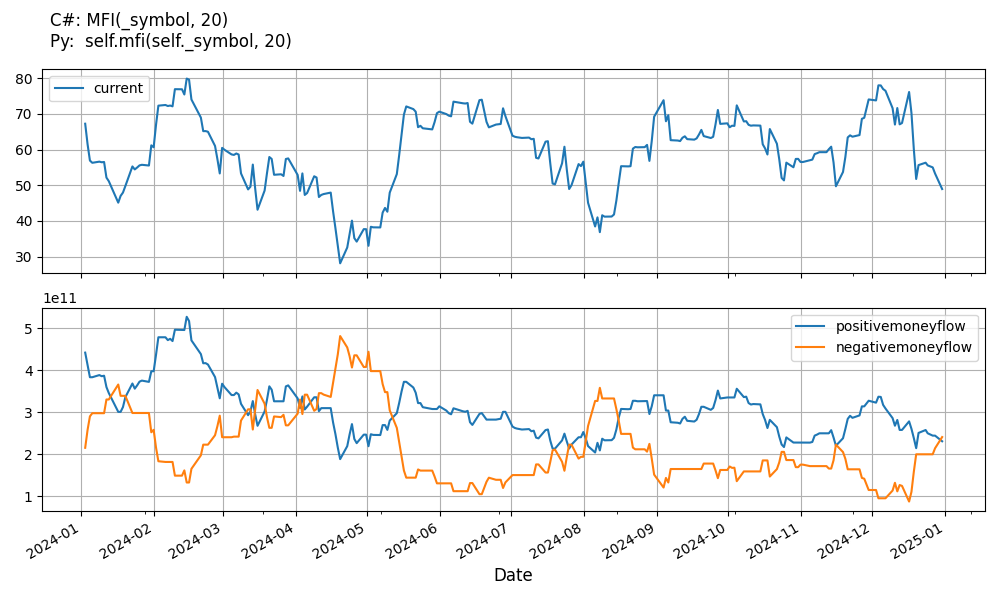# Supported Indicators

## Money Flow Index

### Introduction

The Money Flow Index (MFI) is an oscillator that uses both price and volume to measure buying and selling pressure Typical Price = (High + Low + Close)/3 Money Flow = Typical Price x Volume Positive Money Flow = Sum of the money flows of all days where the typical price is greater than the previous day's typical price Negative Money Flow = Sum of the money flows of all days where the typical price is less than the previous day's typical price Money Flow Ratio = (14-period Positive Money Flow)/(14-period Negative Money Flow) Money Flow Index = 100 x Positive Money Flow / ( Positive Money Flow + Negative Money Flow)

To view the implementation of this indicator, see the LEAN GitHub repository.

### Using MFI Indicator

To create an automatic indicators for MoneyFlowIndex, call the MFI helper method from the QCAlgorithm class. The MFI method creates a MoneyFlowIndex object, hooks it up for automatic updates, and returns it so you can used it in your algorithm. In most cases, you should call the helper method in the Initialize method.

public class MoneyFlowIndexAlgorithm : QCAlgorithm
{
private Symbol _symbol;
private MoneyFlowIndex _mfi;

public override void Initialize()
{
_mfi = MFI(_symbol, 20);
}

public override void OnData(Slice data)
{
{
// The current value of _mfi is represented by itself (_mfi)
// or _mfi.Current.Value
Plot("MoneyFlowIndex", "mfi", _mfi);
// Plot all properties of mfi
Plot("MoneyFlowIndex", "positivemoneyflow", _mfi.PositiveMoneyFlow);
Plot("MoneyFlowIndex", "negativemoneyflow", _mfi.NegativeMoneyFlow);
Plot("MoneyFlowIndex", "previoustypicalprice", _mfi.PreviousTypicalPrice);
}
}
}
class MoneyFlowIndexAlgorithm(QCAlgorithm):
def Initialize(self) -> None:
self.mfi = self.MFI(self.symbol, 20)

def OnData(self, slice: Slice) -> None:
# The current value of self.mfi is represented by self.mfi.Current.Value
self.Plot("MoneyFlowIndex", "mfi", self.mfi.Current.Value)
# Plot all attributes of self.mfi
self.Plot("MoneyFlowIndex", "positivemoneyflow", self.mfi.PositiveMoneyFlow.Current.Value)
self.Plot("MoneyFlowIndex", "negativemoneyflow", self.mfi.NegativeMoneyFlow.Current.Value)
self.Plot("MoneyFlowIndex", "previoustypicalprice", self.mfi.PreviousTypicalPrice)


The following reference table describes the MFI method:

### MFI()1/1

            MoneyFlowIndex QuantConnect.Algorithm.QCAlgorithm.MFI (
Symbol                            symbol,
Int32                             period,
*Nullable<Resolution>       resolution,
*Func<IBaseData, TradeBar>  selector
)


Creates a new MoneyFlowIndex indicator. The indicator will be automatically updated on the given resolution.

If you don't provide a resolution, it defaults to the security resolution. If you provide a resolution, it must be greater than or equal to the resolution of the security. For instance, if you subscribe to hourly data for a security, you should update its indicator with data that spans 1 hour or longer.

You can manually create a MoneyFlowIndex indicator, so it doesn't automatically update. Manual indicators let you update their values with any data you choose.

Updating your indicator manually enables you to control when the indicator is updated and what data you use to update it. To manually update the indicator, call the Update method with a TradeBar. The indicator will only be ready after you prime it with enough data.

public class MoneyFlowIndexAlgorithm : QCAlgorithm
{
private Symbol _symbol;
private MoneyFlowIndex _mfi;

public override void Initialize()
{
_mfi = new MoneyFlowIndex(20);
}

public override void OnData(Slice data)
{
if (data.Bars.TryGeValue(_symbol, out var bar))
{
_mfi.Update(bar);
}

{
// The current value of _mfi is represented by itself (_mfi)
// or _mfi.Current.Value
Plot("MoneyFlowIndex", "mfi", _mfi);
// Plot all properties of mfi
Plot("MoneyFlowIndex", "positivemoneyflow", _mfi.PositiveMoneyFlow);
Plot("MoneyFlowIndex", "negativemoneyflow", _mfi.NegativeMoneyFlow);
Plot("MoneyFlowIndex", "previoustypicalprice", _mfi.PreviousTypicalPrice);
}
}
}
class MoneyFlowIndexAlgorithm(QCAlgorithm):
def Initialize(self) -> None:
self.mfi = MoneyFlowIndex(20)

def OnData(self, slice: Slice) -> None:
bar = slice.Bars.get(self.symbol)
if bar:
self.mfi.Update(bar)

# The current value of self.mfi is represented by self.mfi.Current.Value
self.Plot("MoneyFlowIndex", "mfi", self.mfi.Current.Value)
# Plot all attributes of self.mfi
self.Plot("MoneyFlowIndex", "positivemoneyflow", self.mfi.PositiveMoneyFlow.Current.Value)
self.Plot("MoneyFlowIndex", "negativemoneyflow", self.mfi.NegativeMoneyFlow.Current.Value)
self.Plot("MoneyFlowIndex", "previoustypicalprice", self.mfi.PreviousTypicalPrice)


To register a manual indicator for automatic updates with the security data, call the RegisterIndicator method.

public class MoneyFlowIndexAlgorithm : QCAlgorithm
{
private Symbol _symbol;
private MoneyFlowIndex _mfi;

public override void Initialize()
{
_mfi = new MoneyFlowIndex(20);
RegisterIndicator(_symbol, _mfi, Resolution.Daily);
}

public override void OnData(Slice data)
{
{
// The current value of _mfi is represented by itself (_mfi)
// or _mfi.Current.Value
Plot("MoneyFlowIndex", "mfi", _mfi);
// Plot all properties of mfi
Plot("MoneyFlowIndex", "positivemoneyflow", _mfi.PositiveMoneyFlow);
Plot("MoneyFlowIndex", "negativemoneyflow", _mfi.NegativeMoneyFlow);
}
}
}
class MoneyFlowIndexAlgorithm(QCAlgorithm):
def Initialize(self) -> None:
self.mfi = MoneyFlowIndex(20)
self.RegisterIndicator(self.symbol, self.mfi, Resolution.Daily)

def OnData(self, slice: Slice) -> None:
# The current value of self.mfi is represented by self.mfi.Current.Value
self.Plot("MoneyFlowIndex", "mfi", self.mfi.Current.Value)
# Plot all attributes of self.mfi
self.Plot("MoneyFlowIndex", "positivemoneyflow", self.mfi.PositiveMoneyFlow.Current.Value)
self.Plot("MoneyFlowIndex", "negativemoneyflow", self.mfi.NegativeMoneyFlow.Current.Value)


The following reference table describes the MoneyFlowIndex constructor:

### MoneyFlowIndex()1/2

            MoneyFlowIndex QuantConnect.Indicators.MoneyFlowIndex (
int  period
)


Initializes a new instance of the MoneyFlowIndex class.

### MoneyFlowIndex()2/2

            MoneyFlowIndex QuantConnect.Indicators.MoneyFlowIndex (
string  name,
int     period
)


Initializes a new instance of the MoneyFlowIndex class.

### Visualization

The following image shows plot values of selected properties of MoneyFlowIndex using the plotly library.You can also see our Videos. You can also get in touch with us via Discord.